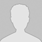#学习小专题

## 有机物分子式的确定

(1)该化合物分子中各元素原子的数目(N)之比为

N(C)：N(H)=80％/12 ：20％/1=1 ：3

(2)设该化合物分子中含有n个CH3，则：

N=Mr(A)/ Mr(CH3)=30/15=2

N(C)=30×80%/12=2

N(H)=30×20%/1=6

C：C         CO2

12        44

M(C)    44g・mol－1×0.1mol

H：2H         H2O

2          18

M(H)     2.7g

m(C)+m(H)=1.2g＋0.3g=1.5g＜2.3g

(2)求该有机物的相对分子质量：

Mr(A)=d・Mr(空气)=1.6×29=46

(3)求该有机物分子中各元素原子的数目：

Mr(A)=Mr(空气)×1.6=46

n(A)=m(A)/M(A)=2.3g/46g・mol－1=0.05mol

C：n(C)=n(CO2)=0.1mol       m(C)=1.2g

H：n(H)=n(H2O)=2×2.7g/18g・mol－1=0.3mol       m(H)=0.3g

O：n(O)=(2.3g－1.2g－0.3g)/16 g・mol－1=0.05mol

n(A)：n(C)：n(H)：n(O)=0.05mol:0.1mol:0.3mol:0.05mol=1:2:6:1

Mr(A)= Mr(空气)×1.6=46

n(A)=m(A)/M(A)=2.3g/46g・mol－1=0.05mol

n(CO2)=0.1mol      n(H2O)= 2.7g/18g・mol－1=0.15mol

CxHyOz＋(xy/4－z/2) O2=== xCO2＋y/2 H2O

1mol                                       x            y/2

0.05mol                                0.1mol     0.15mol

x=2y=6

A分子式为C2H6O

2CnH2n＋1OH2Na===2CnH2n＋1ONa＋H2

2mol×m(A)                                         22.4L

0.60g                                               0.112L

CnH2nOm＋2O2=== nCO2nH2O

2n/(1＋2)=4/3       n=2        m=4＋2－4=2

Mr(混)=13×2=26

m=n×M(混)=0.2mol×26g・mol－1=5.2g

14n g・mol－1×x=2.8g

14n g・mol－1×x＋16 g・mol－1(0.2mol－x)=5.2

x=0.05mol        n=4

(1)求某种气体的相对分子质量的途径主要有两种：

①已知标准状况下气体密度：Mr=22.4ρ

②已知一种气体对另一种气体的相对密度：Mr1=Mr2・ρ12

(2)求有机物实验式途径主要有两种：

①直接由分子中各元素质量分数求各原子的个数比。

②由燃烧产物二氧化碳求碳原子数，由氢原子数，由反应物质量和碳氢质量确定是否有氧元素及其原子个数。

(3)确定有机物分子式主有以下四条途径：

①已知实验式和相对分子质量

②已知通式和相对分子质量

③已知混合物分子的平均组成和通式

④已知反应方程式和反应的相关量

(责任编辑：化学自习室)

------分隔线----------------------------

------分隔线----------------------------

 从有机物的结构简式推导计算分子 微课视频之酷学习：有机物分子式 微课视频： 有机物分子式的推断 仅由有机物的相对分子质量求分子 微课视频：有机物分子式的推断及 有机物化学式及有机物分子结构鉴 如何根据有机物的结构简式快速判 烃的化学式求法汇总 巧用“转换法”速解有机题 有机物分子式的求解方法 有机物化学式的确定 确定有机物分子组成的重要规律 有机物分子式确定的基本思路与方 如何根据有机物的结构简式快速 有机物化学式的确定方法 从有机物中各组成元素的质量分数 如何根据有机物的结构简式快速写 有机化学确定烃分子式的常用方法• 全部评论（0
还没有评论，快来抢沙发吧！